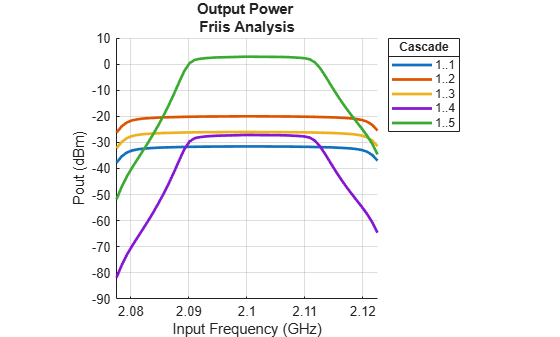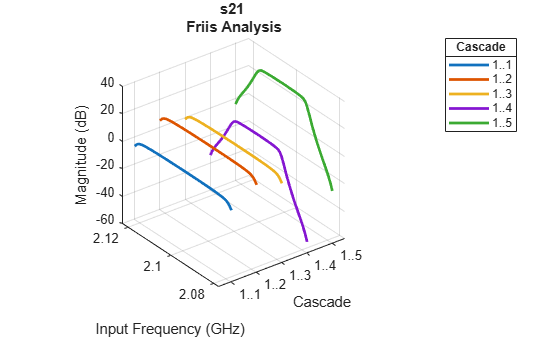# rfplot

Plot cumulative RF budget result versus cascade input frequency

## Syntax

``rfplot(rfobj)``
``rfplot(rfobj,rfpara)``
``rfplot(rfobj,m,n)``
``rfplot(ax,___)``

## Description

````rfplot(rfobj)` plots the magnitude response of `S-Parameters`, S21 for the cascaded budget object, `rfobj`.```

example

````rfplot(rfobj,rfpara)` plots the RF budget result specified by RF parameters `rfpara` versus a range of input frequencies. The input frequencies are applied to the cascade of elements in the RF budget object, `rfobj`.Cumulative (that is, terminated subcascade) results are automatically computed to show the variation of the RF budget result through the entire design. ```
````rfplot(rfobj,m,n)` plots the magnitude response of `S-Parameters`, Smn (S11, S12, S21 , or S22) for the cascaded budget object, `rfobj`.```
````rfplot(ax,___)` plots the cumulative RF budget result on the axes specified in `ax` instead of the current axes. Specify `ax` as the first input argument followed by any of the input argument combinations in the previous syntaxes. Return the current axes using the `gca` function.```

## Examples

collapse all

Create an RF system.

Create an RF bandpass filter using the Touchstone® file `RFBudget_RF`.

`f1 = nport('RFBudget_RF.s2p','RFBandpassFilter');`

Create an amplifier with a gain of 11.53 dB, a noise figure (NF) of 1.53 dB, and an output third-order intercept (OIP3) of 35 dBm.

`a1 = amplifier(Name='RFAmplifier',Gain=11.53,NF=1.53,OIP3=35);`

Create a demodulator with a gain of –6 dB, a NF of 4 dB, and an OIP3 of 50 dBm.

```d = modulator(Name='Demodulator',Gain=-6,NF=4,OIP3=50, ... LO=2.03e9,ConverterType='Down');```

Create an IF bandpass filter using the Touchstone file `RFBudget_IF`.

`f2 = nport('RFBudget_IF.s2p','IFBandpassFilter');`

Create an amplifier with a gain of 30 dB, a NF of 8 dB, and an OIP3 of 37 dBm.

`a2 = amplifier(Name='IFAmplifier',Gain=30,NF=8,OIP3=37);`

Calculate the RF budget of the system using an input frequency of 2.1 GHz, an input power of –30 dBm, and a bandwidth of 45 MHz.

`b = rfbudget([f1 a1 d f2 a2],2.1e9,-30,45e6)`
```b = rfbudget with properties: Elements: [1x5 rf.internal.rfbudget.Element] InputFrequency: 2.1 GHz AvailableInputPower: -30 dBm SignalBandwidth: 45 MHz Solver: Friis AutoUpdate: true Analysis Results OutputFrequency: (GHz) [ 2.1 2.1 0.07 0.07 0.07] OutputPower: (dBm) [-31.53 -20 -26 -27.15 2.847] TransducerGain: (dB) [-1.534 9.996 3.996 2.847 32.85] NF: (dB) [ 1.533 3.064 3.377 3.611 7.036] IIP2: (dBm) [] OIP2: (dBm) [] IIP3: (dBm) [ Inf 25 24.97 24.97 4.116] OIP3: (dBm) [ Inf 35 28.97 27.82 36.96] SNR: (dB) [ 65.91 64.38 64.07 63.83 60.41] ```

Plot the available output power.

```rfplot(b,'Pout') view(90,0)```Plot the transducer gain.

```rfplot(b,'GainT') view(90,0)```Plot S-parameters of an RF system on a Smith Chart and a Polar plot.

`s = smithplot(b,1,1,'GridType','ZY'); ``p = polar(b,2,1); `Create an amplifier with a gain of 4 dB.

`a = amplifier(Gain=4);`

Create a modulator with an OIP3 of 13 dBm.

`m = modulator(OIP3=13);`

Create an N-port element using `passive.s2p`.

`n = nport('passive.s2p');`

Create an RF element with a gain of 10 dB.

`r = rfelement(Gain=10);`

Calculate the RF budget of a series of RF elements at an input frequency of 2.1 GHz, an available input power of –30 dB, and a bandwidth of 10 MHz.

`b = rfbudget([a m r n],2.1e9,-30,10e6);`

Show the analysis in the RF plot.

`rfplot(b)`Group Delay

To plot the group delay, first plot the S11 data for the RF System.

`rfplot(b,1,1)`Use the `Group Delay `option on the plot graph to plot the group delay of the RF system.Phase Delay

Use the `Phase Delay` option on the plot graph to plot the phase delay of the RF System.## Input Arguments

collapse all

RF budget object, specified as a `rfbudget` object.

Example: `rfplot(rfobj,'Pout')`

RF parameters, specified as one of the following:

• `'Pout'` - Available output power (dBm)

• `'GainT'` - Transducer gain (dB)

• `'NF'` - Noise Figure (dB)

• `'OIP3'` - Output Third-Order Intercept (dBm)

• `'IIP3`' - Input Third-Order Intercept (dBm)

• `'SNR'` - Signal-to-Noise Ratio (dB)

• `'Sparameters'` - S - Parameters S21 magnitude response (dB)

Example: `rfplot(rfobj,'Pout')` where `'Pout'` is the available output power of an RF system obtained from the RF budget analysis.

Axes object, specified as an `axes` or a `uiaxes` object.

## Version History

Introduced in R2017b